# Inversion of a matrix

(diff) ← Older revision | Latest revision (diff) | Newer revision → (diff)

An algorithm applicable for the numerical computation of an inverse matrix. As for the solution of linear systems, methods for numerical inversion can be subdivided into direct and iterative methods; however, iterative methods play a considerably smaller role here because of their laboriousness.

Most of the direct methods for the inversion of a matrix are based on the idea of decomposing the given matrix into easily invertible factors. If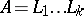is such a decomposition, thenThe Jordan method (see ) is a typical representative of the direct methods (and one of the most generally used).

Letbe a non-singular matrix of order. The construction of the inverse matrixproceeds insteps; the result of the-th step is a matrixwhose firstcolumns are the same as the corresponding columns of the identity matrix. The passage from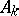(let) to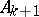from a matrix point of view is equivalent to left multiplication ofby a matrixwhich differs from the identity matrix only in the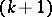-st column. The elements of this column are chosen in order to reduce the-st column ofto the identity, and they have the form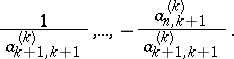From the relations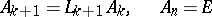it follows thatand(1)

To obtain the factorization (1) for the inverse matrix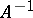requires aboutmultiplications and about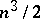additions. Approximately the same number of operations is necessary to multiply out the matrices in (1) to obtain the explicit form for. In many applications of matrix inversion the use of (1) is just as satisfactory as that of the explicit form. For example, the computation of the product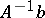, whereis a column vector, requires the same arithmetical work in both cases. The memory requirements when implemented on a computer are also the same.

In the above description of the Jordan method it was assumed for simplicity that all the elements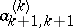(called the pivots) were non-zero. In reality the Jordan method, like the methods of Gauss type for the solution of linear systems (cf. also Gauss method), is usually applied with one or another scheme for choosing the pivots. The use of such a scheme is equivalent to introducing additional factors in (1) which take account of the permutations of the rows and columns of the inverse matrix. The accuracy of the computed solution, as in the case of linear systems, depends on the rate of growth of the matrix entries in the intermediate steps of the method. Such growth, with subsequent deterioration of the accuracy in the computed solution, is more probable in the Jordan method, even with choice of pivots, than in methods of Gauss type.

The matrix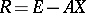is called the residual corresponding to the approximate inversefor. One has the estimateThus, the norm of the residual is a bound for the relative precision of the approximate inverse matrix. This is an important difference between the problem of numerical inversion of a matrix and the solution of linear systems, where (for example, in orthogonal methods or methods of Gauss type) the residual is usually small, and the quality of the solution obtained depends on the conditioning of the system.

The inversion of several important classes of matrices can be achieved by methods that are significantly more economical than in the general case. Such classes include Toeplitz, Hankel, banded (and in particular tri-diagonal) matrices, block matrices having a Toeplitz structure or the structure of Kronecker products, etc. For example, letbe a Toeplitz matrix of orderwith entries fromor: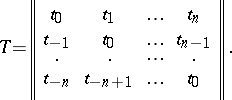Assume that not only, but also its principal submatrices of orderare non-singular. Then the matrix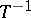, which, in general, is already not Toeplitz, has the representation (see ):(2)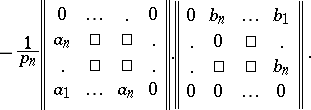Here the vectorsare, respectively, the first and last column of. Thus,is completely described by giving its first and last columns. From (2) all the elements ofcan be successively computed: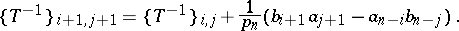This computation requires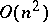arithmetical operations.

In economic algorithms for the inversion of Toeplitz matrices (see , for example) the computation of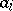,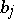and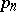is performed by recurrence formulas and also requiresoperations. The condition that the principal submatrices are non-singular can be relaxed, while still needing onlyarithmetical operations.

Matrix inversion is sometimes used in order to solve linear systems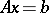by the formula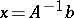. For matrices of general form such a procedure makes little sense, since it is accompanied by an increase in arithmetical work and loss of numerical stability as compared to direct solution of the linear system. But for Toeplitz (and related) matrices the situation is different. As the representation (2) shows, the computation of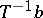reduces to the performance of four multiplications of Toeplitz matrices by vectors and a subtraction of vectors. There are economic algorithms for the multiplication of Toeplitz matrices by vectors, requiring (for order)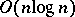operations. Such asymptotic behaviour of the number of arithmetical operations is not yet attainable for the solution of Toeplitz systems (currently, the best methods for these require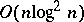operations). Hence, for the repeated solution of linear systemswith the same Toeplitz matrixand varying right-hand sides, a preliminary inversion ofseems reasonable.

Preliminary matrix inversion can be justified in the case of repeated solution of linear systems with the same matrix of general form on a computer with a large number of parallel processors. The reason is that, as compared to the multiplication of a matrix by a vector, direct methods for solving linear systems do not have such convenient parallelization.

In many cases (for example in the quasi-Newton methods of mathematical programming) it is required to invert a matrixthat differs from a matrixwith known inverse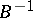by a matrix of rank 1 or (in the case of symmetric matrix) by a symmetric matrix of rank 2. Such a reconstruction of an inverse matrix can be carried out inoperations for matrices of order. The following formula may serve as an example (see ): Ifandare column vectors, then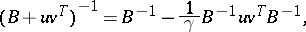where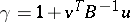is assumed to be non-zero.

From the point of view of the theory of computational complexity, the problem of matrix inversion has complexity of the same order (on a sequential machine) as the problem of solving a linear system (if certain natural conditions on the rate of growth of complexity of both problems as their order increases are satisfied ). This complexity has order not exceeding.

How to Cite This Entry:
Inversion of a matrix. Encyclopedia of Mathematics. URL: http://encyclopediaofmath.org/index.php?title=Inversion_of_a_matrix&oldid=11899
This article was adapted from an original article by Kh.D. Ikramov (originator), which appeared in Encyclopedia of Mathematics - ISBN 1402006098. See original article